# RS Aggarwal Solutions Chapter 16 Coordinate Geometry Exercise 16B Class 10 Maths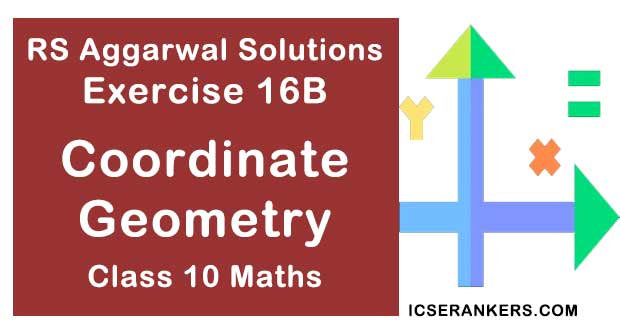Chapter Name RS Aggarwal Chapter 16 Coordinate Geometry Book Name RS Aggarwal Mathematics for Class 10 Other Exercises Exercise 16AExercise 16CExercise 16DMCQ Related Study NCERT Solutions for Class 10 Maths

### Exercise 16B Solutions

1. Find the coordinates of the point which divides the join of A(-1. 7) and B(4, -3) in the ratio 2 : 3

Solution

The end points of AB are A(-1, 7) and B(4, -3)

Therefore (x1 = -1, y1 = 7) and (x2 = 4, y2 = -3)

Also, m = 2 and n = 3

Let the required point be P(x, y)

By section formula, we get

x = (mx2 + nx1)/(m + n), y = (my2 + ny1)/(m + n)

⇒ x = {2 × 4 + 3 × (-1)}/(2 + 3), y = {2 × (-3) + 3 × 7}/(2 + 3)

⇒ x = (8 – 3)/5, y = (-6 + 21)/5

⇒ x = 5/5, y = 15/5

Therefore, x = 1 and y = 3

Hence, the coordinates of the required point are (1, 3).

2. Find the co-ordinates of the point which divides the join of A(-5, 11) and B(4, -7) in the ratio 7: 2.

Solution

The end points of AB are A(-5, 11) and B(4, -7)

Therefore, (x1 = - 5, y1 = 11) and (x2 = 4, y2 = -7)

Also, m = 7 and n = 2

Let the required point be P(x, y).

By section formula, we get

x = (mx2 + nx1)/(m + n), y = (my2 + ny1)/(m + n)

⇒ x = {7 × 4 + 2 × (-5)}/(7 + 2), y = {7 × (-7) + 2 × 11}/(7 + 2)

⇒ x = (28 – 10)/9, y = (-49 + 22)/9

⇒ x = 18/9, y = -(27/9)

Therefore, x = 2 and y = - 3

Hence, the required point are P(2, -3).

3. If the coordinates of points A and B are (-2, -2) and (2, -4) respectively. Find the coordinates of the point P such that AP = 3/7 AB, where P lies on the segment AB.

Solution

The coordinates of the points A and B are (-2, -2) and (2, -4) respectively, where

AP = 3/7 AB and P lies on the line segment AB. So

AP + BP = AB

⇒ AP + BP = 7AP/3 (∵ AP = 3/7 AB)

⇒ BP = 7AP/3 – AP

⇒ AP/BP = 3/4

Let (x, y) be the coordinates of P which divides AB in the ratio 3 : 4 internally. Then

x = {(3 × 2 + 4 × (-2)}/(3 + 4) = (6 – 8)/7 = -(2/7)

y = {3 × (-4) + 4 × (-2)}/(3 + 4) = (-12 – 18)/7 = -(20/7)

Hence, the coordinates of point P are (- 2/7, - 20/7).

4. Point A lies on the line segment PQ joining P(6, -6) and Q(-4, 1) in such a way that PA/PQ = 2/5. If that point A also lies on the line 3x + k(y + 1) = 0 find the value of k.

Solution

Let the coordinates of A be (x, y). Here PA/PQ = 2/5 so,

PA + AQ = PQ

⇒ PA + AQ = 5PA/2 [∵ PA = 2/5 PQ)

⇒ AQ = 5PA/2 – PA

⇒ AQ/PA = 3/2

⇒ PA/AQ = 2/3

Let (x, y) be the coordinates of A, which dives PQ in the ratio 2 : 3 internally, Then using section formula, we get

x = {(2 × (-4) + 3 × (6)}/(2 + 3) = ( -8 + 18)/5 = 10/5 = 2

y = {2 × (-1) + 3 × (-6)}/(2 + 3) = (-2 -18)/5 = -20/5 = - 4

Now, the point (2, -4) lies on the line 3x + k(y + 1) = 0, therefore

3 × 2 + k (-4 + 1) = 0

⇒ 3k = 6

⇒ k = 6/3 = 2

Hence, k = 2.

5. Points P, Q, R and S divide the line segment joining the points A(1, 2) and B(6, 7) in five equal parts. Find the coordinates of the points P, Q and R.

Solution

Since, the points P, Q, R and S divide the line segment joining the points A(1, 2) and B(6, 7) in five equal parts, so

AP = PQ = QR = R = SB

Here, point P divides AB in the ratio of 1 : 4 internally. So using section formula, we get

Coordinates of P = {(1 × (6) + 4 × (1)}/(1 + 4), {(1 × (7) + 4 × (2)}/(1 + 4)

= (6 + 4)/5, (7 + 8)/5

= (2, 3)

The point Q divides AB in the ratio of 2 : 3 internally. So using section formula, we get

Coordinates of Q = {(2 × (6) + 3 × (1)}/(2 + 3), {(2 × (7) + 3 × (2)}/(2 + 3)

= (12 + 3)/5, (14 + 6)/5

= (3, 4)

The point R divides AB in the ratio of 3 : 2 internally So using section formula, we get

Coordinates of R = (3 × (6) + 2 × (1)/(3 + 2) , (3 × (7) + 2 × (2)}/(3 + 2)

= (18 + 2)/5, (21 + 4)/5

= (4, 5)

Hence, the coordinates of the points P, Q and R are (2, 3), (3, 4) and (4, 5) respectively.

6. Points P, Q and R in that order are dividing line segment joining A(1, 6) and B(5, -2) in four equal parts. Find the coordinates of P, Q and R.

Solution

The given points are A(1, 6) and B(5, -2)

Then P(x, y) is a point that devices the line AB in the ratio 1 : 3

By the section formula:

x = (mx2 + nx1)/(m + n), y = (my1 + ny1)/(m + n)

⇒ x = (1 × 5 + 3 × 1)/(1 + 3), y = (1 × (-2) + 3 × 6)/(1 + 3)

⇒ x = (5 + 3)/4, y = (-2 + 18)/4

⇒ x = 8/4, y = 16/4

⇒ x = 2 and y = 4

Therefore, the coordinates of point P are (2, 4)

Let Q be the mid-point of AB

Then, Q (x, y)

x = (x1 + x2)/2, y = (y1 + y2)/2

⇒ x = (1 + 5)/2, y = (6 + (-2)/2

⇒ x = 6/2, y = 4/2

⇒ x = 3, y = 2

Therefore, the coordinates of Q are (3, 2)

Let R(x, y) be a point that divides AB in the ratio 3 : 1

Then, by the section formula:

x = (mx2 + nx1)/(m + n) , y = (my2 + ny1)/(m + n)

⇒ x = (3 × 5 + 1 × 1)/(3 + 1), y = (3 × (-2) + 1 × 6)/(3 + 1)

⇒ x = (15 + 1)/4, y = (3 × (-2) + 1 × 6)/(3 + 2)

⇒ x = (15 + 1)/4, y = (-6 + 6)/4

⇒ x = 16/4, y = 0/4

⇒ x = 4 and y = 0

Therefore, the coordinates of R are (4, 0).

Hence, the coordinates of point P,Q and Rare (2, 4), (3, 2) and (4, 0) respectively.

7. The line segment joining the points A(3, -4) and B(1, 2) is trisected at the points P(p, -2) and Q(5/3, q). Find the values of p and q.

Solution

Let P and Q b the points of trisection of AB

Then P divides AB in the ratio 1 : 2

So, the coordinates of P are

x = (mx2 + nx1)/(m + n), y = (my2 + ny1)/(m + n)

⇒ x = (1 × 1 + 2 × (3)}/(1 + 2), y = {1 × 2 + 2 × (-4)}/(1 + 2)

⇒ x = (1 + 6)/3, y = (2 – 8)/3

⇒ x = 7/3, y = -(6/3)

⇒ x = 7/3, y = -2

Hence, the coordinates of Pare (7/3, -2)

But, (p, -2) are the coordinates of P.

So, p = 7/3

Also, Q divides the line AB in the ratio 2 : 1

So, the coordinates of Q are

x = (mx2 + mx1)/(m + n), y = (my2 + my1)/(m + n)

⇒ x = (2 × 1 + 1 × 3)/(2 + 1), y = {2 × 2 + 1 × (-4)}/(2 + 1)

⇒ x = (2 + 3)/3, y = (4 – 4)/3

⇒ x = 5/3, y = 0

Hence, Coordinates of Q are (5/3, 0)

But the given coordinates of Q are (5/3, q)

So, q = 0

Thus, p = 7/3 and q = 0

8. Find the coordinates of the midpoints of the line segment joining

(i) A(3, 0) and B(-5, 4)

(ii) P(-11, -8) and Q(8, -2)

Solution

(i) The given points are A(3, 0) and B(-5, 4)

Let (x, y) be the midpoint of AB. Then:

x = (x1 + x2)/2, y = (y+ y2)/2

⇒ x = {3 + (-5)}/2, y = (0 + 4)/2

⇒ x = -2/2, y = 4/2

⇒ x = -1, y = 2

Therefore, (-1, 2) are the coordinates of midpoint of AB.

(ii) The given points are P(-11, -8) and Q(8, -2).

Let (x, y) be the midpoint of PQ. Then:

x = (x1 + x2)/2, y = (y1 + y2)/2

x = (-11 + 8)/2, y = (-8 – 2)/2

⇒ x = - (3/2), y = -(10/2)

⇒ x = -(3/2), y = - 5

Therefore, (-3/2, - 5) are the coordinates of midpoint of PQ.

9. If (2, p) is the midpoint of the line segment joining the points A(6, - 5) and B(-2, 11) find the value of p.

Solution

The given points are A(6, - 5) and B(-2, 11)

Let (x, y) be the midpoint of AB. Then,

x = (x1 + x2)/2, y = (y1 + y2)/2

⇒ x = 6 + (-2)/2, y = (-5 + 11)/2

⇒ x = (6 – 2)/2, y = (- 5 + 11)/2

⇒ x = 4/2, y = 6/2

⇒ x = 2, y = 3

So, the midpoint of AB is (2, 3).

But it is given that midpoint of AB is (2, p).

Therefore, the value of p = 3

10. The midpoint of the line segment joining A(2a, 4) and B(-2, 3b) is C(1, 2a + 1). Find the values of and b.

Solution

The points are A(2a, 4) and B(-2, 3b)

Let C(1, 2a + 1) be the mid-point of AB. Then:

x = (x1 + x2)/2, y = (y1 + y2)/2

⇒ 1 = (2a (-2)/2, 2a + 1 = (4 + 3b)/2

⇒ 2 = 2a – 2, 4a + 2 = 4 + 3b

⇒ 2a = 2 + 2, 4a – 3b = 4 – 2

⇒ a = 4/2, 4a – 3b = 2

⇒ a = 2, 4a – 3b = 2

Putting the value of a in the equation 4a + 3b = 2, we get:

4(2) – 3b = 2

⇒ - 3b = 2 – 8 = - 6

⇒ b = 6/3 = 2

Therefore, a = 2 and b = 2

11. The line segment joining A(-2, 9) and B(6, 3) is a diameter of a circle with center C. Find the coordinates of C.

Solution

The given point are A(-2, 9) and B(6, 3)

Then, C(x, y) is the midpoint of AB.

x = (x1 + x2)/2, y = (y1 + y2)/2

⇒ x = (-2 + 6)/2, y = (9 + 3)/2

⇒ x = 4/2, y = 12/2

⇒ x = 2, y = 6

Therefore, the coordinates of point C are (2, 6).

12. Find the coordinates of a point A, where AB is a diameter of a circle with center C(2, -3) and the other end of the diameter is B (1, 4).

Solution

C(2, -3) is the center of the given circle. Let A(a, b) and B(1, 4) be the two end-points of the given diameter AB. Then, the coordinates of C are

x = (a + 1)/2, y = (b + 4)/2

It is given that x = 2 and y = - 3

⇒ 2 = (a + 1)/2, - 3 = (b + 4)/2

⇒ 4 = a + 1, - 6 = b + 4

⇒ a = 4 – 1, b = - 6 – 4

⇒ a = 3, b = - 10

Therefore, the coordinates of point A are (3, -10).

13. In what ratio does the point P(2, 5) divide the join of A (8, 2) and B(- 6, 9) ?

Solution

Let the point P(2, 5) divides AB in the ratio k : 1.

Then, by section formula, the coordinates of P are x = (-6k + 8)/(k + 1), y = (9k + 2)/(k + 1)

It is given that the coordinates of P are (2, 5)

⇒ 2 = (-6k + 8)/(k + 1), 5 = (9k + 2)/(k + 1)

⇒ 2k + 2 = -6k + 8, 5k + 5 = 9k + 2

⇒ 2k + 6k = 8 – 2, 5 – 2 = 9k – 5k

⇒ 8k = 6, 4k = 3

⇒ k = 6/8, k = 3/4

⇒ k = 3/4 in each case.

Therefore, the point P(2, 5) divides AB in the ratio 3 : 4.

14. Find the ratio in which the point P(3/4, 5/12) divides the line segment joining the points A(1/2, 3/2) and B(2, -5).

Solution

Let k : 1 be the ratio in which the point P(3/4, 5/12) divides the line segment joining the points A(1/2, 3/2) and (2, -5). Then

(3/4, 5/12) = {k(2) + 1/2/(k + 1), k(-5) + 2/2)/(k + 1)}

⇒ {k(2) + 1/2}/(k + 1) = 3/4 and {k(-5) + 3/2}/(k + 1) = 5/12

⇒ 8k + 2 = 3k + 3 and – 60k + 18 = 5k + 5

⇒ k = 1/5 and k = 1/5

Hence, required ratio is 1 : 5.

15. Find the ratio in which the point P(m, 6) divides the join of A(-4, 3) and B(2, 8). Also, find the value of m.

Solution

Let the point P(m, 6) divide the line AB in the ratio k : 1.

Then, by the section formula:

x = (mx2 + nx1)/(m + n), y = (my2 + ny1)/(m + n)

The coordinates of P are (m, 6).

m = (2k – 4)/(k + 1), 6 = (8k + 3)/(k + 1)

⇒ m(k + 1) = 2k – 4, 6k + 6 = 8k + 3

⇒ m(k + 1) = 2k – 4, 6 – 3 = 8k – 6k

⇒ m(k + 1)= 2k – 4, 2k = 3

⇒ m(k + 1) = 2k – 4, k = 3/2

Therefore, the point P divides the line AB in the ratio 3 : 2.

Now, putting the value of k in the equation m(k + 1) = 2k – 4, we get:

m(3/2 + 1) = 2(3/2) – 4

⇒ m (3 + 2)/2 = 3 – 4

⇒ 5m/2 = - 1

⇒ 5m = -2

⇒ m = -(2/5)

Therefore, the value of m = -(2/5)

So, the coordinates of P are (-2/5, 6).

16. Find the ratio in which the print (-3, k) divide the join of A(-5, -4) and B(-2, 3). Also, find the value of k.

Solution

Let the point P(-3, k) divide the line AB in the ratio s : 1

Then, by the section formula:

x = (mx1 + nx1)/(m + n), y = (my2 + ny1)/(m + n)

The coordinates of P are (-3, k).

-3 = (-2s – 5)/(s + 1) , k = (3s – 4)/(s + 1)

⇒ - 3s – 3 = - 2s – 5, k(s + 1) = 3s – 4

⇒ - 3s + 2s = - 5 + 3, k(s + 1) = 3s – 4

⇒ - s = - 2, k(s + 1) = 3s – 4

⇒ s = 2, k(s + 1) = 3s – 4

Therefore, the point P divides the line AB in the ratio 2 : 1.

Now, Putting the value of s in the equation k(s + 1) = 3s – 4, we get:

k(2 + 1) = 3(2) – 4

⇒ 3k = 6 – 4

⇒ 3k = 2

⇒ k = 2/3

Therefore, the value of k = 2/3

That is, the coordinates of P are (-3, 2/3).

17. In what ratio is the line segment joining A(2, -3) and B(5, 6) divide by the x-axis ? Also, find the coordinates of the point of division.

Solution

Let AB be divided by the x-axis in the ratio k : 1 at the point P.

Then, by section formula the coordinates of P are

P = (5k + 2)/(k + 1), (6k – 3)/(k + 1)

But P lies on the x-axis, so, its ordinate is 0.

Therefore, (6k – 3)/|(k + 1) = 0

⇒ 6k – 3 = 0

⇒ 6k = 3

⇒ k = 3/6

⇒ k = 1/2

Therefore, the required ratio is 1/2 : 1, which is same as 1 : 2.

Thus, the x-axis divides the line AB lie in the ratio 1 : 2 at the point P.

Applying k = 1/2, we get the coordinates of point,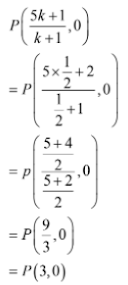Hence, the point of intersection of AB and the x-axis is P(3, 0)

18. In what ratio is the line segment joining the points A(-2, -3) and B(3, 7) divided by the y-axis? Also, find the coordinates of the point of division.

Solution

Let AB be divided by the x-axis in the ratio k : 1 at the point P.

Then, by section formula the coordinates of P are

P = {(3k – 2)/(k + 1), (7k – 3)/(k + 1)}

But P lies on the y-axis; so, its abscissa is 0.

Therefore, the required ratio is 2/3 : 1, which is same as 2 : 3

Thus, the x-axis divides the line AB in the ratio 2 : 3 at the point P.

Applying k = 2/3, we get the coordinates of point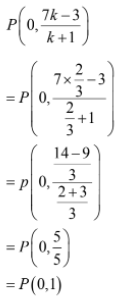Hence, the point of intersection of AB and the x-axis is P(0, 1).

19. In what ratio does the line x – y – 2 = 0 divide the line segment joining the points A(3, -1) and B(8, 9) ?

Solution

Let the line x – y – 2 = 0 divide the line segment joining the points A(3, -1) and B(8, 9) in the ratio k : 1 at P.

Then, the coordinates of P are

P(8k + 3)/(k + 1), (9k – 1)/(k + 1)

Since, P lies on the line x – y – 2 = 0, we have:

(8k + 3)/(k + 1) – (9k – 1)/(k + 1) – 2 = 0

⇒ 8k + 3 – 9k + 1 – 2k – 2 = 0

⇒ 8k – 9k – 2k + 3 + 1 – 2 = 0

⇒ - 3k + 2 = 0

⇒ -3k = -2

⇒ k = 2/3

So, the required ratio is 2/3 : 1, which is equal to 2 : 3.

20. Find the lengths of the medians of a ABC whose vertices are A(0, -1), B(2, 1) and C(0, 3).

Solution

The vertices of △ABC are A(0, -1), B(2, 1) and C(0, 3).

Let AD, BE and CF be the medians of △ABC.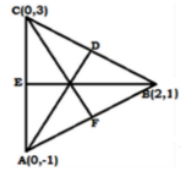Let D be the midpoint of BC. So, the coordinates of D are

D(2 + 0)/2, (1 + 3)/2 i.e., D(2/2, 4/2) i.e., D(1, 2)

Let E be the midpoint of AC. So the coordinate of E are

E(0 + 0)/2, (-1 + 3)/2 i.e., E(0/2, 0/2) i.e, E(0, 1)

Let F be the midpoint of AB. So the coordinates of F are

F(0 + 2)/2, (- 1 + 1)/2 i.e., F(2/2, 0/2) i.e., F(1, 0)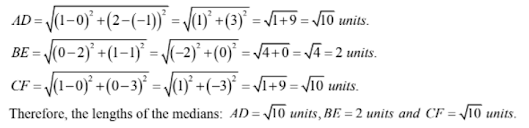21. Find the centroid of ABC whose vertices are A(-1, 0), B(5, -2) and C (8, 2)

Solution

Here, (x1 = -1, y1 = 0), (x2 = 5, y2 = -2) and (x3 = 8, y3 = 2)

Let G (x, y) be the centroid of the △ABC. Then,

x = 1/3(x1 + x2 + x3) = 1/3(-1 + 5 + 8) = 1/3(12) = 4

y = 1/3(y1 + y2 + y3) = 1/3(0 – 2 + 2) = 1/3(0) = 0

Hence, the centroid of △ABC is G(4, 0).

22. If G(-2, 1) is the centroid of a ABC and two of its vertices are A(1, -6) and B(-5, 2), find the third vertex of the triangle.

Solution

Two vertices of △ABC are A(1, -6) and B(-5, 2).

Let the third vertex be C(a, b).

Then, the coordinates of its centroid are

C(1 – 5 + a)/3, (-6 + 2 + b)/3)

C(-4 + a)/3, (-4 + b)/3

But it is given that G(-2, 1) is the centroid. Therefore,

-2 = (-4 + a)/3, 1 = (-4 + b)/3

⇒ 6 = - 4 + a, 3 = - 4 + b

⇒ - 6 + 4 = a, 3 + 4 = b

⇒ a = - 2, b = 7

Therefore, the third vertex of △ABC is C(-2, 7).

23. Find the third vertex of a ABC if two of its vertices are B(-3, 1) and C(0, -2), and its centroid is at the origin.

Solution

Two vertices of △ABC are B(-3, 1) and C(0, -2). Let the third vertex be A(a, b).

Then, the coordinates of its centroid are

(-3 + 0 + a)/3, (1 – 2 + b)/2

i.e., (-3 + a)/3, (-1 + b)/3)

But it is given that centroid is at the origin, that is G(0, 0). Therefore,

0 = (-3 + a)/3, 0 = (-1 + b)/3

⇒ 0 = - 3 + a, 0 = -1 + b

⇒ 3 = a, 1 = b

⇒ a = 3, b = 1

Therefore, the third vertex of △ABC is A(3, 1).

24. Show that the points A(3, 1), B(0, -2), C(1, 1) and D(4, 4) are the vertices of parallelogram ABCD.

Solution

The points are A(3, 1), B(0, -2), C(1, 1) and D(4, 4)

Join AC and BD, intersecting at O.We know that the diagonals of a parallelogram bisect each other.

Midpoint of AC = (3 + 1)/2, (1 + 1)/2 = (4/2, 2/2) = (2, 1)

Midpoint of BD = (0 + 4)/2, (-2 + 4)/2 = (4/2, 2/2) = (2, 1)

Thus, the diagonals AC and BD have the same midpoint

Therefore, ABCD is a parallelogram.

25. If the points P(a, -11) , Q(5, b), R(2, 15) and S(1, 1) are the vertices of a parallelogram PQRS, find the values of a and b.

Solution

The points are P(a, -11), Q(5, b), R(2, 15) and S(1, 1).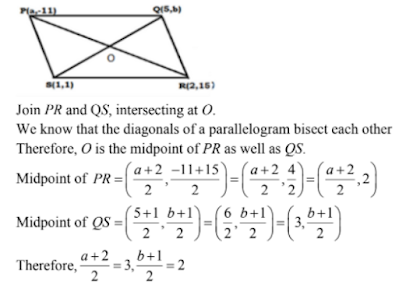⇒ a + 2 = 6, b + 1 = 4

⇒ a = 6 – 2, b = 4 – 1

⇒ a = 4 and b = 3

26. If three consecutive vertices of a parallelogram ABCD are A(1, -2), B(3, 6) and C(5, 10). Find its fourth vertex D.

Solution

Let A(1, -2), B(3, 6) and C(5, 10) be the three vertices of a parallelogram ABCD and the fourth vertex be D(a, b).

Join AC and BD intersecting at O.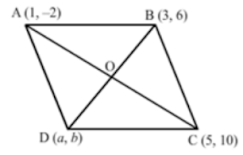We know that the diagonals of a parallelogram bisect each other

Therefore, O is the midpoint of AC as well as BD.

Midpoint of AC = (1 + 5)/2, (-2 + 10)/2 = (6/2, 8/2) = (3, 4)

Midpoint of BD = {(3 + a)/2, (6 + b)/2}

Therefore, (3 + a)/2 = 3 and (6 + b)/2 = 4

⇒ 3 + a = 6 and 6 + b = 8

⇒ a = 6 – 3 and b = 8 – 6

⇒ a = 3 and b = 2.

Therefore, the fourth vertex is D(3, 2).

27. In what ratio does y-axis divide the line segment joining the points (-4, 7) and (3 -7)?

Solution

Let y-axis divides the line segment joining the points (-4, 7) and (3, -7) in the ratio k : 1. Then

0 = (3k – 4)/(k + 1)

⇒ 3k = 4

⇒ k = 4/3

Hence, the required ratio is 4 : 3.

28. If the point P(1/2, y) lies on the line segment joining the points A(3, -5) and B(-7, 9) then find the ratio in which P divides AB. Also, find the value of y.

Solution

Let the point P(1/2, y) divides the line segment joining the points A(3, -5) and B(-7, 9) in the ratio k : 1. Then

(1/2, y) = {(k (-7) + 3)/(k + 1), {k(9) – 3)/(k + 1)}

⇒ (-7k + 3)/(k + 1) = 1/2 and (9k – 5)/(k + 1) = y

⇒ k + 1 = -14 k + 6

⇒ k = 1/3

Now, substituting k = 1/3 in (9k – 5)/(k + 1) = y, we get

(9/3 – 5)/(1/3 + 1) = y

⇒ y = (9 – 15)/(1 + 3) = -(3/2)

Hence, required ratio is 1 : 3 and y = -(3/2).

29. Find the ratio which the line segment joining the points A(3, -3) and B(-2, 7) is divided by x-axis. Also, find the point of division.

Solution

The line segment joining the points A(3, - 3) and B(-2, 7) is divided by x-axis. Let the required ratio be k : 1, So,

Now,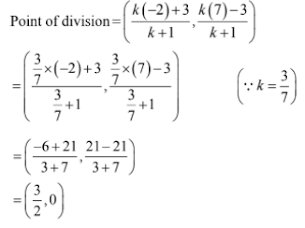Hence, the required ratio is 3 : 7 and the point of division is (3/2, 0)

30. The base QR of an equilateral triangle PQR lies on x-axis. The coordinates of the point Q are (-4, 0) and origin is the midpoint of the base. Find the coordinates of the point P and R.

Solution

Let (x, 0) be the coordinates of R. Then

0 = (-4 + x)/2

⇒ x = 4

Thus, the coordinates of R are (4, 0).

Here PQ = QR = PR and the coordinates of P lies on y-axis. Let the coordinates of P be (0, y). Then,

PQ = QR

⇒ PQ2 = QR2

⇒ (0 + 4)2 + (y + 0)2 = 82

⇒ y2 = 64 – 16 = 48

⇒ y = ±4√3

Hence, the required coordinates are R(4, 0) and P(0, 4√3) or P(0, -4√3).

31. The base BC of an equilateral triangle ABC lies on y-axis. The coordinates of point C are (0, -3). The origin is the midpoint of the base. Find the coordinates of the points A and B. Also, find the coordinates of another point D such that ABCD is a rhombus.

Solution

Let (0, y) be the coordinates of B. Then

0 = (-3 + y)/2

⇒ y = 3

Thus, the coordinates of B are (0, 3)

Here, AB = BC = AC and by symmetry the coordinates of A lies on x-axis.

Let the coordinates of A be (x, 0), Then

AB = BC

⇒ AB2 = BC2

⇒ (x – 0)2 + (0 – 3)= 62

⇒ x2 = 36 – 9 = 27

⇒ x = ±3√3

If the coordinates of point A are (3, √3, 0) then the coordinates of D are (-3√3, 0).

If the coordinates of point A are (-3√3, 0) then the coordinates of D are (-3√3, 0)

Hence, the required coordinates are A(3√3, 0), B(0, 3) and D(-3√3, 0) or A(3√3, 0) and D(-3√3, 0) or A(-3√3, 0), B(0, 3) and D(3√3, 0)

32. Find the ratio in which the point (-1, y) lying on the line segment joining points A(-3, 10) and (6, -8) divides it. Also, find the value of y.

Solution

Let k be the ratio in which P(-1, y) divides the line segment joining the points

A(-3, 10) and B(6, -8)

Then,

(-1, y) = {(k(6) – 3/(k + 1), (k(-8) + 10)/(k + 1)}

⇒ k(6) – 3}/(k + 1) = - 1 and y = {(k(-8) + 10}/(k + 1)

⇒ k = 2/7

Substituting k = 2/7 in y = {k(-8) + 10}/(k + 1), we get

y = {(-8 × 2)/7 + 10}/(2/7 + 1)

= (-16 + 70)/9

= 6

33. ABCD is rectangle formed by points A(-1, -1), B(-1, 4), C(5, 4) and D(5, -1). If P, Q, R and S be the midpoints of AB, BC, CD and DA respectively, Show that PQRS is a rhombus.

Solution

Here the points P, Q, R and S are the midpoint of AB, BC, CD and DA respectively. Then,

Coordinates of P = (-1 – 1)/2, (-1 + 4)/2 = (-1, 3/2)

Coordinates of Q = (-1 + 5)/2, (4 + 4)/2 = (2, 4)

Coordinates of R = (5 + 5)/2, (4 – 1)/2 = (5, 3/2)

Coordinates of S = (- 1 + 5)/2, (-1 – 1)/2 = (2, -1)

Now,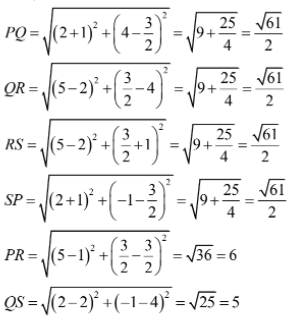Thus, PQ = QR = RS = SP and PR ≠ QS therefore PQRS is a rhombus.

34. The midpoint P of the line segment joining points A(-10, 4 and B(-2, 0) lies on the line segment joining the points C(-9, -4) and D(-4, y). Find the ratio in which P divides CD. Also, find the value of y.

Solution

The midpoint of AB is (-10 – 2)/2, (4 + 10)/2 = P(-6, 2)

Let k be the ratio in which P divides CD. So,

(-6, 2) = {k(-4) – 9}/(k + 1), {k(y) – 4)/(k + 1)

⇒ k(-4) – 9}/(k + 1) = - 6 and k(y) – 4}/(k + 1) = 2

⇒ k = 3/2

Now, substituting k = 3/2 in k(y) – 4}/(k + 1) = 2, we get

{y × 3/2 – 4}/(3/2 + 1) = 2

⇒ (3y – 8)/5 = 2

⇒ y = (10 + 8)/3 = 6

Hence, the required ratio is 3 : 2 and y = 6.